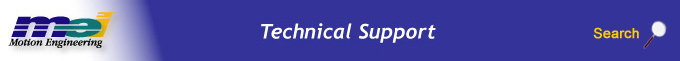.

MEIMotorEncoderModulo

Definition

 `typedef struct MEIMotorEncoderModulo { MPI_BOOL reverse; /* set to TRUE if external encoder is already moduloed at a non 32 bit boundary */ long value;} MEIMotorEncoderModulo;` Change History: Added in the 03.04.00.

Description

The controller can modulo incomming encoder feedback. In cases where the you want to keep track of how many times the motor has passed a certain position (ex: how many times a motor has gone through one revolution), the modulo function can be used. If you want to keep track of how many times a motor has gone through one revolution, set the modulo value to the number of encoder counts per revolution and set reverse to FALSE. The lower 32 bits of the motor feedback will represent the position within one revolution of the motor. The upper 32 bits will represent how many times the motor has gone through one revolution.

For motors that do not have 32 bits of feedback, the reverse modulo function MUST be used. Set the modulo value equal to the encoder resolution and set reverse to TRUE. The controller will create a 64-bit encoder position in the motor object from the encoder feedback.

 reverse If the encoder provides 32 bits of feedback, set the reverse field to FALSE. In the case where an encoder does not provide 32 bits of feedback, the reverse field is used. For example, if an encoder has 24 bits of feedback, set the modulo value to 224 and set the reverse field to TRUE. The controller will create a full 64-bit encoder position based on the 24-bit feedback from the motor. value Set to the Modulo value. Modulo is always active. The modulo value can be any number from 0 to 4294967295 (232-1). A value of 0 (default) is equivalent to 232 (0x100000000) and causes a rollover at 32 bits. The 64-bit motor feedback value is created from the 32-bit position feedback from the motor. Modulo is generally used on encoders that provide 32 bits of feedback and the user needs to keep track of the number of times a certain value is exceeded. For example, a motor with 2000 counts/rev could use a modulo value of 2000 so that the number of revolutions is counted. In this case, the upper 32 bits of the motor feedback value represents the number of revolutions and the lower 32 bits of the motor feedback value represents the position (0 – 1999) of the motor within one revolution. In the case where an encoder does not provide 32 bits of feedback, the reverse field is used. For example, if an encoder has 24 bits of feedback, set the modulo value to 224 and set the reverse field to TRUE. The controller will create a full 64-bit encoder position based on the 24-bit feedback from the motor.